# [Dot Product] Vector Proection

• Highway

#### Highway

[Dot Product] Vector Projection

## Homework Statement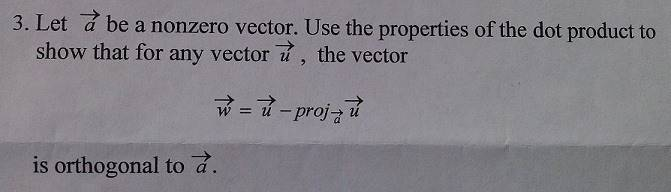## Homework Equations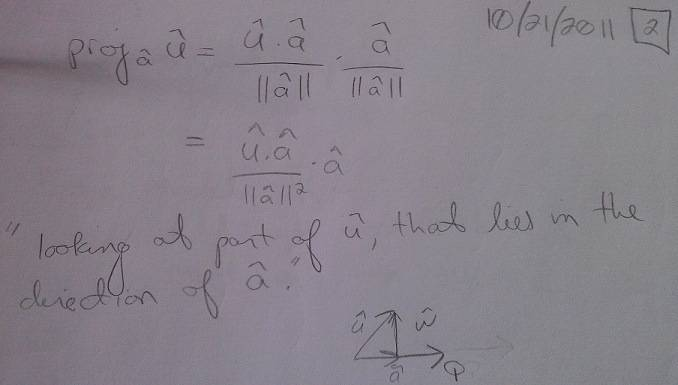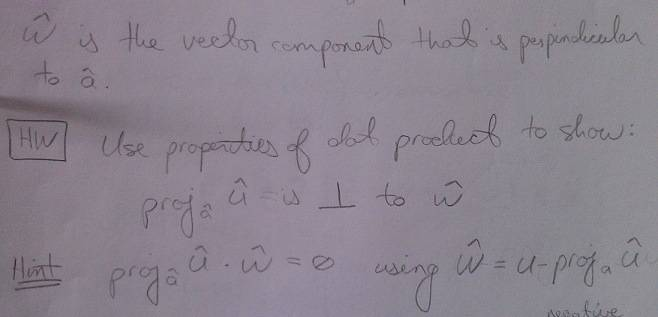## The Attempt at a Solution

I am not sure what to do here -- I know that the projection of u onto a "dotted" with w = 0 by definition, but I don't know how to show this.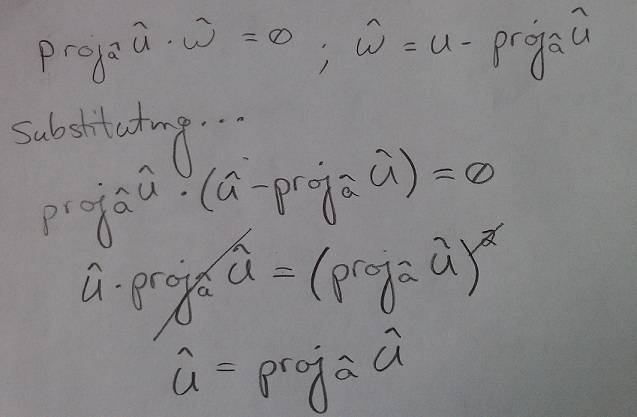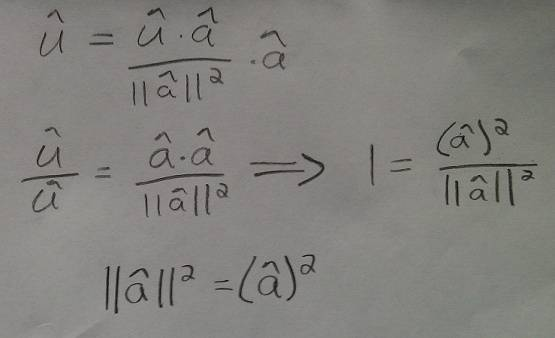added this second part after plugging in for the definition of the projection we derived in class, then simplified. . .

Last edited:
anyone?

Hi Highway!You have an expression for the projection.
Can you substitute that (and only that) in the formula you have for w?

To show that 2 vectors are orthogonal, you need to show that their dot product is zero. That is, that $\vec a \cdot \vec w = 0$.

What you need to know, is that there are calculation rules for dot products.
For instance $\vec a \cdot (\vec b+\vec c) = \vec a \cdot \vec b + \vec a \cdot \vec c$.

Can you simplify the expression for $\vec a \cdot \vec w = 0$?

Hi Highway!You have an expression for the projection.
Can you substitute that (and only that) in the formula you have for w?

To show that 2 vectors are orthogonal, you need to show that their dot product is zero. That is, that $\vec a \cdot \vec w = 0$.

What you need to know, is that there are calculation rules for dot products.
For instance $\vec a \cdot (\vec b+\vec c) = \vec a \cdot \vec b + \vec a \cdot \vec c$.

Can you simplify the expression for $\vec a \cdot \vec w = 0$?

Thanks! I got it figured out :P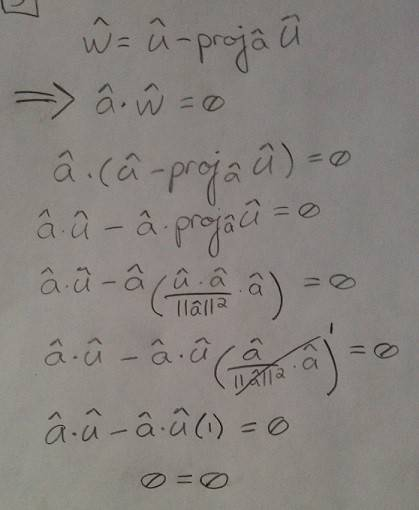Congrats!